# Lower Cost-Market - BC Bookkeeping Tutorials|dwmbeancounter.comTitle
Go to content

## Lower Cost-MarketLower Of Cost Or Market Rule

Up until now, we've been using Cost to calculate the value of our Ending Inventory. In some instances, because we bean counter types want to be more conservative, we depart from cost and value our inventory at less than cost. We use a rule called the Lower Of Cost Or Market. The rule was created to account for the loss of potential inventory value due to changes such as price and obsolescence.

When would such a rule be used ? Let's assume our product Super Widgets cost us \$5.00 a unit at the beginning of the year and our normal Selling Price is \$10.00. Let's also assume at the end of the year we can purchase our Super Widgets for \$1.00 per unit. If we can purchase them for \$1.00 a piece, so can our competitors. What do you think is more than likely to happen to our \$10.00 selling price ? Our competitors could easily sell the Super Widgets for \$5.00 a unit or even less in effect forcing us to reduce our price in order to be able to sell our Super Widgets.

We depart from the cost basis when the value (utility ) of the goods is no longer as great as its cost. In other words, we suffered a loss. If I can now buy Super Widgets at \$1.00 (replacement cost) instead of \$5.00, the goods I have on hand are no longer worth the \$5.00 that I originally paid for them.

The term Market (market price) means the cost of replacing the goods at the inventory date. In our earlier example, \$1.00 is the market value or replacement cost. The rule is based on the assumption that a decline in the market or replacement cost will normally result in a reduction of the selling price and thus a decline in the value of our inventory.

Normally, the loss (write down) is reflected in the Cost Of Goods Sold Account. If however, a decline in market value is substantial and unusual (material) , it must be recorded in a separate Loss Account such as Loss Due to Market Decline.

The Lower Of Cost Or Market Rule is used along with our selected Inventory Method (Periodic, Perpetual, or Retail) and in conjunction with any of the Costing Methods that we discussed for assigning costs except LIFO. It can be used in conjunction with the FIFO, Weighted Average, or Specific Identification Costing Methods (Flows) that we discussed in earlier lessons. Why not LIFO ? It's kind of like when we were kids and our parents said we couldn't do something and we asked why and they answered "because we said so". In a nutshell, for us living in the United States, the Internal Revenue Service (IRS) says we can't. Other countries may have their own requirements.

The rule may be applied to each (1) product (item-to-item), (2)product groups, departments, or lines , or (3) in total. The rule is normally applied on an item-to-item basis because once again the IRS says so. In addition, it also provides the most conservative valuation of inventory because decreases in the value of one product are not offset by increases in the value of another product.

The Lower Of Cost are Market Rule does have some special requirements that must be used in determining Market Value (replacement cost).

Terminology:

• Cost means the original cost of the inventory.
• Market means current replacement cost (CRC) subject to an upper (ceiling) and lower (floor) limit.
• Net Realizable Value is the current selling price less estimated costs of completion and disposal.
• Net Realizable Value Less Normal Profit is the Net Realizable Value Less an allowance for a Normal Profit Margin.
Note:Normal Profit Percentage is the normal Gross Profit Percent for the product.
• Ceiling-Net Realizable Value (NRV)
• Floor-Net Realizable Value Less Normal Profit Percentage (NRV-Normal Profit)

Special Rule used to determine market value:

• If the Current Replacement Cost (CRC) is greater than the upper limit (ceiling), then use the ceiling (NRV) as market.
• If the Current Replacement Cost (CRC) is below the lower limit (floor), then use the floor Net Realizable Value (NRV) less a normal profit as the market value.
• If the Current Replacement Cost (CRC) is greater than the lower limit (floor) and less than the upper limit (ceiling), then use the Current Replacement Cost as the market value.

We'll use a simple example to help understand the terminology and how the Lower Of Cost or Market Rule is used.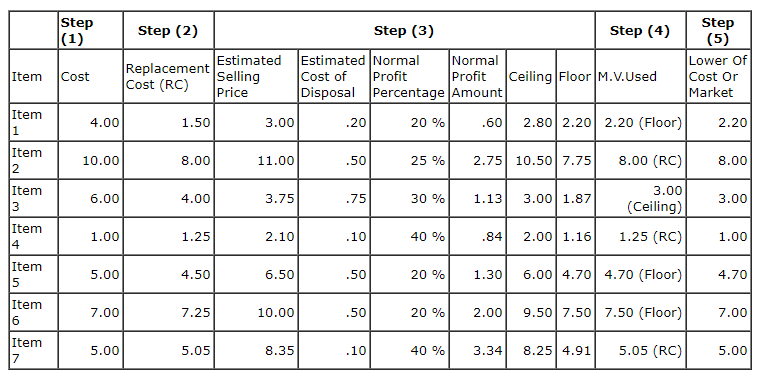Step (1) involves determining the actual cost based on any of the acceptable actual cost methods.

Step (2) involves determining the current Replacement Cost as one of the values used to determine the market value used in the calculation.

Step(3) calculates the other two values, the floor and the ceiling, used to determine the market value used in the calculation.

Step (4) compares the three values of Market Value (replacement cost, the ceiling, and the floor) and uses the special rules to arrive at the market value that is used to compare to the Actual Cost of the item.

Step (5) simply compares the appropriate market value (replacement cost, the ceiling, or the floor) and the actual cost and selects the lower of the two amounts.

The ceiling and floor ensure that excessive profits or losses are not recognized in future periods. The ceiling protects against recognizing excessive losses, while the floor protects against recognizing excessive profits in future periods. Without the ceiling, products could possibly be assigned a value greater than their sales value. Without the floor, inventories could be assigned a value less than their net realizable value less a normal profit.

Let's check out some of the calculations and the reasoning behind some of the calculations presented in our table of products above.

• Item 1
Ceiling Calculation:
Ceiling = Estimated Selling Price - Costs Of Disposal
Ceiling = \$3.00 - .20
Ceiling = \$2.80

Floor Calculation:
Floor = Estimated Selling Price - Costs Of Disposal - Normal Profit
Normal Profit = Normal Profit Margin x Estimated Selling Price
Normal Profit = .20 x \$3.00
Normal Profit = .60
Floor = \$3.00 - .20 - .60
Floor = \$2.20

Using our special rule, since our current replacement cost \$1.50 is less than our floor amount of \$2.20, we use our floor amount of \$2.20 as the market value to compare with our actual cost.

Since our market value \$2.20 is lower than our actual cost of \$4.00, we use the market value of \$2.20 to value our ending inventory of Item 1.

• Item 2
Ceiling Calculation:
Ceiling = Estimated Selling Price - Costs Of Disposal
Ceiling = \$11.00 - .50
Ceiling = \$10.50

Floor Calculation:
Floor = Estimated Selling Price - Costs Of Disposal - Normal Profit
Normal Profit = Normal Profit Margin x Estimated Selling Price
Normal Profit = .25 x \$11.00
Normal Profit = \$2.75
Floor = \$11.00 - .50 - 2.75
Floor = \$7.75

Using our special rule, since our current replacement cost \$8.00 is greater than our floor amount of \$7.75 and less than our ceiling amount of \$10.50 we use our current replacement cost of \$8.00 as the market value to compare with our actual cost.

Since our market value \$8.00 is lower than our actual cost of \$10.00, we use the market value of \$8.00 to value our ending inventory of Item 2.

• Item 3
Ceiling Calculation:
Ceiling = Estimated Selling Price - Costs Of Disposal
Ceiling = \$3.75 - .75
Ceiling = \$3.00

Floor Calculation:
Floor = Estimated Selling Price - Costs Of Disposal - Normal Profit
Normal Profit = Normal Profit Margin x Estimated Selling Price
Normal Profit = .30 x \$3.75
Normal Profit = \$1.13
Floor = \$3.75 - .50 - 1.13
Floor = \$1.87

Using our special rule, since our current replacement cost \$4.00 is greater than our ceiling amount of \$3.00, we use our ceiling amount of \$3.00 as the market value to compare with our actual cost.

Since our market value \$3.00 is lower than our actual cost of \$6.00, we use the market value of \$3.00 to value our ending inventory of Item 3.

Did you notice, that based on our special rules, each of our three items (products) used a different value for market value ?

• Item 1 used the floor amount.
• Item 2 used current replacement cost.
• Item 3 used the ceiling amount.

I'm not going to illustrate the calculations of the ceiling and floor amounts for Item 4 thru Item 7, but as an exercise why not do the calculations yourself based on the illustrations above and see if yours agree with mine.

We will, however, discuss how the value to use for calculating the inventory for Item 4 thru Item 7 was determined.

• Item 4
Using our special rule, since our current replacement cost \$1.25 is greater than our floor amount of \$1.16 and less than our ceiling amount of \$2.00 we use our current replacement cost of \$1.25 as the market value to compare with our actual cost.

Since our actual cost value of \$1.00 is lower than our market value of \$1.25, we use our actual cost value of \$1.00 to value our ending inventory of Item 4.

• Item 5
Using our special rule, since our current replacement cost \$4.50 is less than our floor amount of \$4.70, we use our floor amount of \$4.70 as the market value to compare with our actual cost.

Since our market value \$4.70 is lower than our actual cost of \$5.00, we use the market value of \$4.70 to value our ending inventory of Item 3.

• Item 6
Using our special rule, since our current replacement cost \$7.25 is less than our floor amount of \$7.50, we use our floor amount of \$7.50 as the market value to compare with our actual cost.

Since our actual cost value of \$7.00 is lower than our market value of \$7.50, we use our actual cost value of \$7.00 to value our ending inventory of Item 6.

• Item 7
Using our special rule, since our current replacement cost \$5.05 is greater than our floor amount of \$4.91 and less than our ceiling amount of \$8.25 we use our current replacement cost of \$5.05 as the market value to compare with our actual cost.

Since our actual cost value of \$5.00 is lower than our market value of \$5.05, we use our actual cost value of \$5.00 to value our ending inventory of Item 7.

If you're having any trouble understanding the concept, I'll give you a simple method for selecting the correct market value (replacement cost, ceiling, or floor) to use to compare with actual cost.

Simple Method To Determine Market Value

1. Calculate or obtain your three possible market values:
Replacement Cost
Ceiling
Floor
2. Arrange the market values in increasing order from the lowest amount to the highest amount.
3. Select the middle value to use as your market value

Let's see if it works. We'll use Item 1 in our table as an example.

1. Calculate or obtain your three possible market values:
Replacement Cost- \$1.50
Ceiling- \$2.80
Floor- \$2.20
2. Arrange the market values in increasing order from the lowest amount to the highest amount.
\$1.50     \$2.20     \$2.80
3. Select the middle value to use as your market value
Our market value to use is:\$2.20

All we're actually doing using the Lower Of Cost Or Market Rule is comparing our actual cost amount with estimated current market conditions (market price) and using either our actual cost or our calculated market value, whichever is the lower, for valuing our inventory.

In other words, all we do is determine the figure to use for market subject to our special rules, and then value the inventory at the lower of our cost or market amount.

Observation: Once inventory (products) have been written down to market, that amount becomes "Cost" for determining Lower Of Cost Or Market in future periods.

We learned earlier that the rule may be applied to each (1) product (item-to-item), (2)product groups, departments, or lines , or (3) in total. Normally the rule is applied on an item-to-item basis because the IRS says so. The following example illustrates applying the three different methods.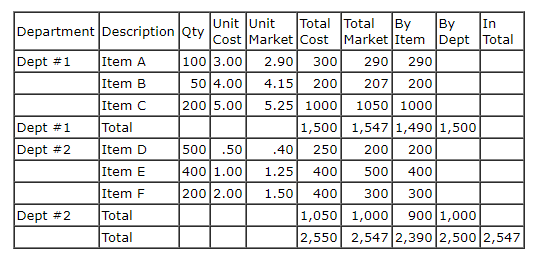In our "little" example our ending inventory values did not vary by a large amount, but we only had six (6) products in our example. What do you think the affect might be if we had a thousand ? The difference could be quite significant.

Individual products produced the lowest value-\$2,390, departments were next lowest-\$2,500, and in total produced the highest amount-\$2,547.

Let's expand our example and prepare an income statement assuming all the amounts are the same except for the value that we'll use for our Ending Inventory. We'll use the different ending inventory values from our previous table.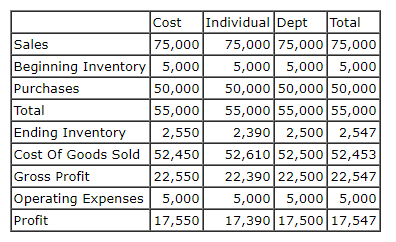Note that all the ending inventory valued using market produced profit results less than actual cost. What method produced the least profit ? The Lower Of Cost applied on an item by item (individual) basis.

You should now be familiar with the calculations and how to use the Lower Of Cost Or Market Rule. Forgot already ? Well let's refresh our memory with the following simple steps.

How to Use the Lower Of Cost or Market Rule• Step (1) -Determine the actual cost values for your ending inventory using any of the acceptable actual cost methods- FIFO, Weighted Average, Specific Identification, or Retail Inventory. We discussed all these methods in earlier lessons. Note:LIFO can not be used.
• Step (2) -Determine our current replacement cost.
• Step (3) -Calculate our market ceiling and floor amounts.
• Step (4) -Arrange our three market values current replacement cost, our ceiling, and our floor in order from the lowest to the highest amount.
• Step (5) -Select the middle market value as the value to use in our final comparison.
• Step (6) -Compare our selected market value with our actual cost amount and use the lower of the two values to calculate our ending inventory amount.

Nothing very complicated, just some time consuming calculations and comparisons.

Last Thought

Before we end our discussion of the Lower Of Cost Or Market Rule, we need to see how the Lower Of Cost Or Market Rule is used with the Retail Inventory Method that we discussed in Lesson 3. In our earlier examples in this lesson, we've been using unit costs and unit market prices. The Retail Inventory by its very nature is known as an aggregate method. We use total costs of inventory and purchases and total selling prices to calculate the value of our ending inventory. So, how do we adjust our calculation so that not only cost but also market value is considered in the calculated results ?

Let's see. Used with the Retail Inventory Method, consideration of the lower of cost or market rule results from the treatment of net markups and net mark downs. An approximation of lower of cost or market is achieved by including net markups and excluding net mark downs from our calculated cost-to-retail ratio.
Let's revisit an example used in Lesson 3 Estimating Inventories. This time we're going to expand our example to illustrate using the Lower Of Cost Or Market Rule with the Retail Inventory Method.

Detailed Example Of Retail Method Calculations considering Lower Of Cost Or Market Rule and assuming FIFO, and Average Cost Flows.

We obtained the following information from our detailed Sales and Accounting Records:

Description                                          Retail               Cost
Sales (Less Discounts)                        \$8,000
Sales Discounts                                      400
Sales Returns                                        200
Breakage                                              100
Markups                                             1,000
Markup Cancellations                             100
Markdowns                                         2,000
Markdown Cancellations                         700
Beginning Inventory                           1,000                700
Purchases                                        10,000             7,500
Purchase Returns                                  500                400

Step (1)
Our first step is to calculate our Goods Available For Sale at Retail. This is done the same way regardless of the Cost Flow Assumption.

Step (2) Calculate our Ending Inventory At Retail by subtracting our Total Retail Value Of Our Goods Sold, Spoiled, or Damaged from our Goods Available For Sale At Retail.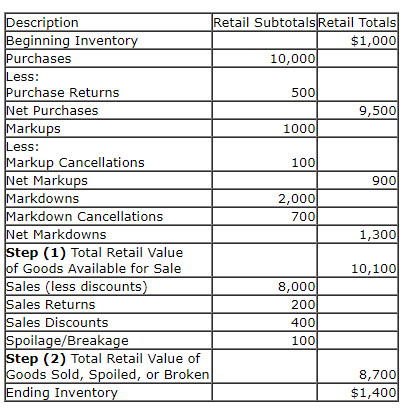Step (3)
Calculate the cost-to-retail ratio to summarize the relationship between cost and retail value. (This ratio is calculated differently for different retail methods.)

Step (4)
Convert the ending inventory stated at retail to ending inventory stated at cost by multiplying by the cost-to-retail ratio.

Two versions assuming Average and FIFO of the retail method are illustrated below. Why didn't we include the LIFO Method in our example ? Easy, the Lower Of Cost Or Market Rule is not allowed with the LIFO method.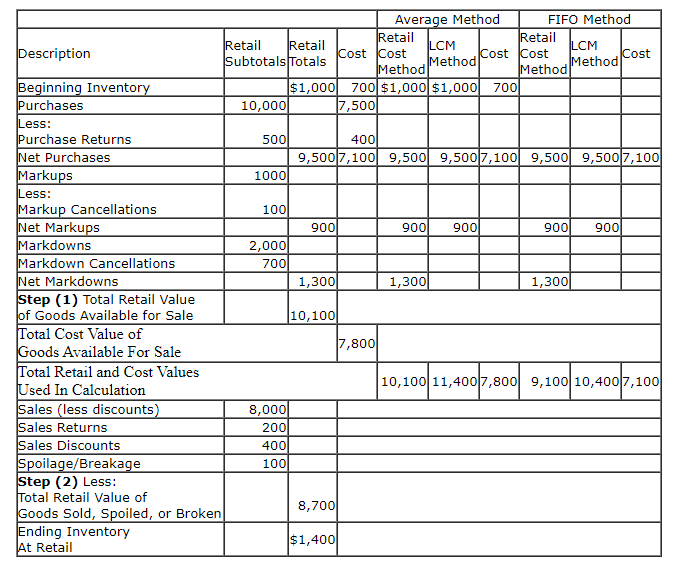Notice the difference between the cost and the lower of cost or market columns for the Average Cost and FIFO methods. Net markups are included and net mark downs are excluded for the lower of cost or market method. Both net markups and net mark downs are included in the "actual" cost method.

Also notice that beginning inventory was omitted in the FIFO calculation and included in the Average calculation.
Step (3)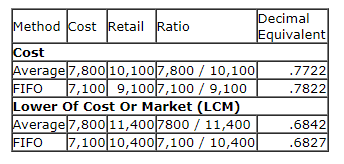Step (4)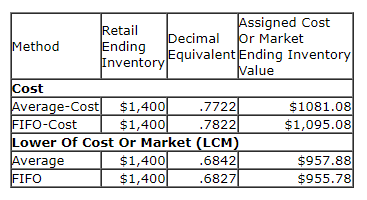Did you notice that the cost-to-retail ratio (decimal equivalent) was lower when the lower of cost or market rule was used ? What affect does this have on our ending inventory and cost of goods sold ? Since the ratio (decimal equivalent) is a smaller number, this means the cost assigned to our ending inventory will be less, resulting in more cost assigned to our cost of goods sold. It logically follows then, that by using the lower of cost or market rule, our amount shown for profit is less.

The reason for including the net markups and excluding the net mark downs using the lower of cost or market rule with the Retail Inventory Method is that this calculation provides the lowest cost-to-retail ratio resulting in a smaller amount of cost assigned to our ending inventory. Let's use a simple example to illustrate.

We'll use the following assumptions:

• One unit of Product A is purchased at a cost of \$10.00 when it's current selling price is \$15.00.
• We're having trouble selling our one unit of Product A for \$15.00 so we markdown our selling price to \$13.
• At year end we still have our one unit of Product A on hand.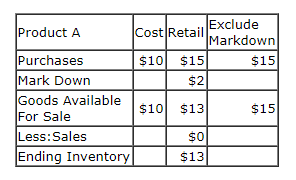Let's calculate our cost-to-retail ratio and ending inventory values.

Including mark downs in our calculation our cost-to-retail ratio is \$10/\$13 and our ending inventory value is 10/13 x \$13 (ending inventory @ retail) or \$10, our original purchases cost.

Excluding mark downs from our calculation our cost-to-retail ratio is \$10/\$15 and our ending inventory value is 10/15 x \$13 (ending inventory @ retail) or \$8.67.

The write down of our inventory from \$10.00 to \$8.67 reflects our loss of utility evidenced by the decline in our selling price from \$15.00 to \$13.00.What's Next ?

Inventory Records
Back to content